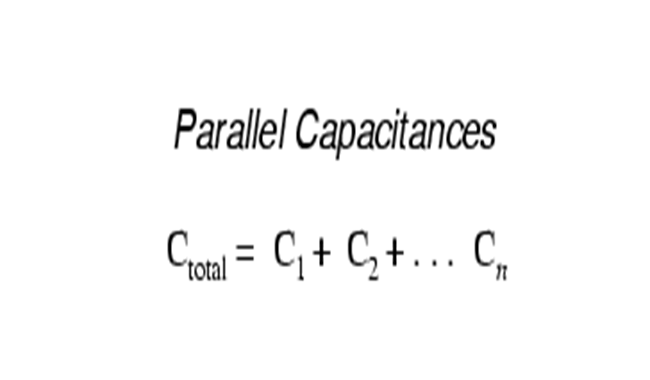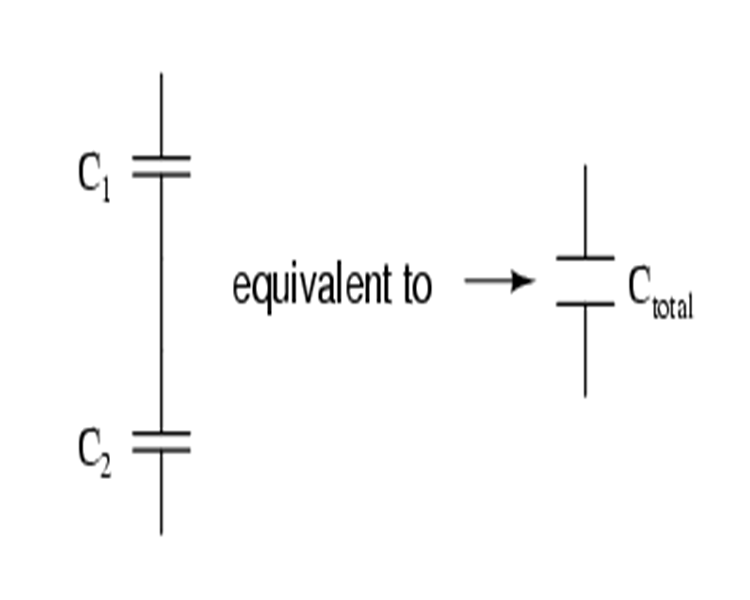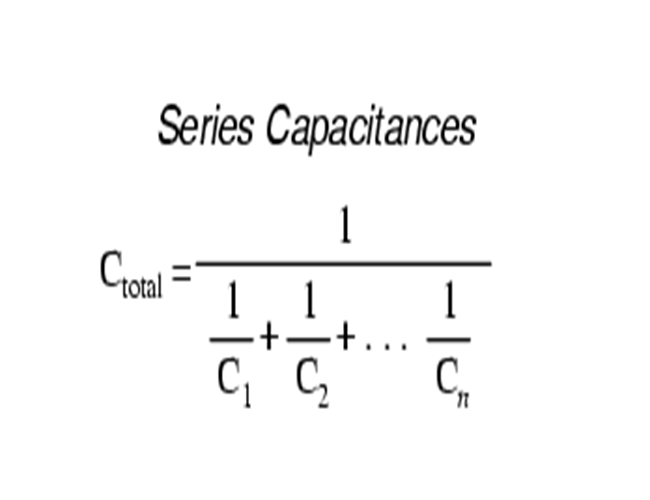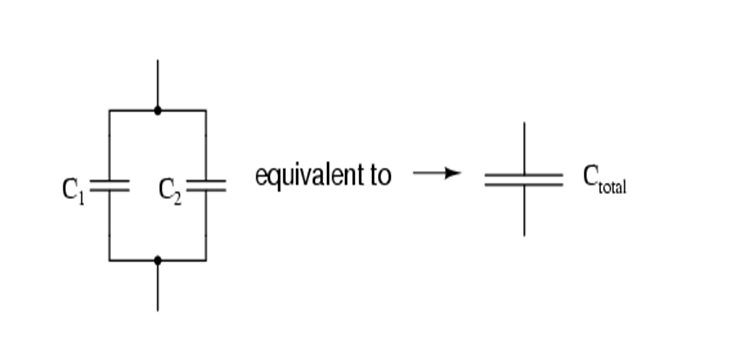Taizhou Dingfeng Electric Appliance Co.,Ltd

## Dingfeng Capacitor---capacitors in series and parallel

publisher: Yani
Time: 2018-04-10capacitors in series and parallel

When capacitors are connected in series, the total capacitance is less than any capacitance of the series capacitor. If two or more capacitors are concatenated together, the overall effect is a (equivalent) capacitor with a single capacitor plate spacing. As all other factors remain unchanged, the increase in plate spacing leads to a decrease in capacitance.Therefore, the total capacitance is less than the capacitance of a single capacitor. The calculation formula of series total capacitance is the same as the calculation formula of parallel resistances:When capacitors are in parallel, the total capacitance is the sum of the capacitors of a single capacitor. If two or more capacitors are connected in parallel, the overall effect is the sum of the total plate area of a single equivalent capacitor. With all other factors unchanged, the increase of plate area leads to the increase of capacitance.Thus, the total capacitance is more than any one of the individual capacitors capacitances. The formula for calculating the parallel total capacitance is the same form as for calculating series resistances:So, this is exactly the opposite of what the resistor shows. In the case of resistance, the concatenation causes the added value to rise, while in parallel, the value decreases. For capacitors, it reverses: parallel leads to added value, and concatenation leads to lower added value.